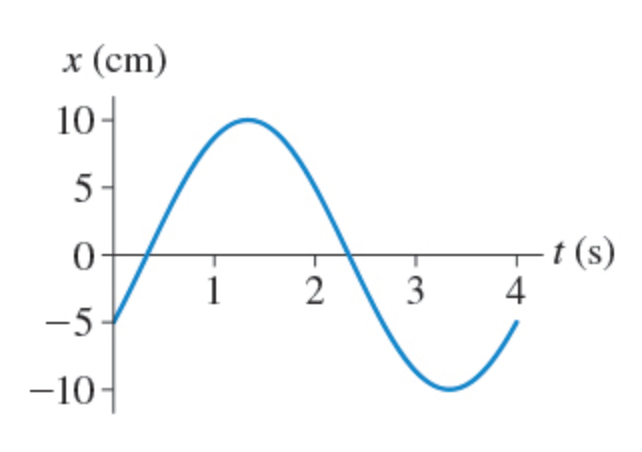# Problem: What is the phase constant of the oscillation shown in the figure? Suppose that −180° ≤ ϕ ≤180°.

###### FREE Expert Solution

General wave solution:

Amplitude, A = 10 cm(1m/100cm) = 0.1 m.

At t = 0s:

ωt = 0

99% (459 ratings)###### Problem DetailsWhat is the phase constant of the oscillation shown in the figure? Suppose that −180° ≤ ϕ ≤180°.